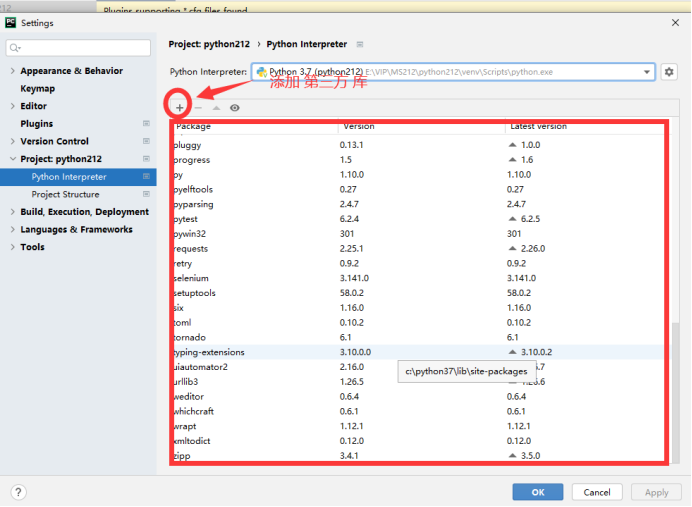# 一、pycharm工具的基本使用

1、本地环境与虚拟环境的区别：不同项目可以共享第三库在虚拟环境

pip install 第三方库包名

pip list

pycharm-setting-project-python interpreter2、pycharm基本常用操作

# 二、python基本语法

1、编码

# -- coding=cp-1252 --

2、标识符

1、第一个字符必须是字母或者下划线

2、标识符的其他部分由字母、数字和下划线组成

3、标识符区分大小写

4、标识符不能是Python保留字

3、python保留字

[‘False’, ‘None’, ‘True’, ‘and’, ‘as’, ‘assert’, ‘async’, ‘await’, ‘break’, ‘class’, ‘continue’, ‘def’, ‘del’, ‘elif’, ‘else’, ‘except’, ‘finally’, ‘for’, ‘from’, ‘global’, ‘if’, ‘import’, ‘in’, ‘is’, ‘lambda’, ‘nonlocal’, ‘not’, ‘or’,
‘pass’, ‘raise’, ‘return’, ‘try’, ‘while’, ‘with’, ‘yield’]

4、注释

5、print函数

print函数：自动化换行输出 等同于 print(字符2,字符2,字符3,…,end=’\n’)

print可以有多个参数 print(字符1,字符2,字符3,…)

print(字符1,字符2,字符3,…,end=’’)

print(“欢迎大家”,end=’\n’) print(“欢迎”,",野马",“Python自动化测试”)

6、行与缩进

7、模块导入

1、import 模块名
2、from 模块名 import 函数名1,函数名2 导入某个模块下指定数据或者函数

# 三、Python常见数据类型

python的变量不需要声明，每个变量在使用前必须赋值，变量赋值了才会被创建

Python常见六大数据类型：
string类型、number类型、tuple类型、list类型、dict类型、set类型

# 四、number类型:数字类型

1、数字类型的定义
num_212class=180
2、查看数字类型的常用函数
type(变量) isinstance(变量名,数据类型)

3、查看变量类型
zx=9.3
print(type(zx))
print(zx)

print(isinstance(num_212class,int))

4、Python数字运算符：+ - / * ** // %
// 商，不一定取整数，它与除数的类型有关系
/ 整除
a**b a的b次幂
num3=3**4
print(num3)
num1=8/3
print(num1)
num2=8//3
print(num2)
num4=8//3.00
print(num4)
ys=8%3
print(“余数：”,ys)

5、常用的数字类型函数
abs()取数字的绝对值
ceil(x)返回数字的上入整数 ceil(4.1) 返回 5
floor(x) 返回数字的下舍整数 floor(5.4) 返回5
round(x,n)四舍五入 n保留几位小数
max() 最大值
min() 最小值
value1=math.floor(5.6)
value2=math.ceil(4.01)
print(value1,value2)

num5=45.89
value3=round(num5)
print(value3)

# 五、Python常见数据类型-string类型（字符串）

1、定义：Python中的字符串用单引号/双引号/三个单/双引号括起来
2、python字符串支持截取 切片

3、特殊的符号

4、字符串运算符：+ * in not in

5、字符串的格式化
string类型
str1=‘欢迎大家’
str2=“哈哈”
str3=""“MS221_NN”""
str4=’’‘MS221_NN’’’
print(type(str1))
print(type(str2))
print(type(str3))
print(type(str4))

print(“学号：”,str3[0:5])

print(str3[-1])

print(str1[-2])
print(str1[-3])

print(str3[1:])

print(str3[0::2])

str4=""“MS221_NN”""
print(str4[-1::-2])
print(str4[::-2])
print(str4[::])

str5=R’\n’
str6=r’\n’
print(str5,str6)
print(“1111”)

Python常用的运算符
+ 字符串的拼接
* 复制 复制2*2
in 成员判断
not in
str7=“abc”
str8=“edfg”
print(str7*3)
print(‘a’ not in str7)

%s 字符串占位符
%d 整数占位符
%u 无符号整型
%f 浮点数字

name=“就这”
age=18
print(“欢迎”+name+",工作年限："+str(age))
print(“格式化方式一：欢迎 %s,工作年限：%d”%(name,age))

str9=“格式化方式二：欢迎{1},工作年限：{0}”.format(age,name)
print(str9)

str10=f"格式化方式三：欢迎{name},工作年限：{age}"
print(str10)